#There are 366 different Starters of The Day, many to choose from. You will find below some starters on the topic of Estimating. A lesson starter does not have to be on the same topic as the main part of the lesson or the topic of the previous lesson. It is often very useful to revise or explore other concepts by using a starter based on a totally different area of Mathematics.

Main Page

### Estimating Starters:Work out which aircraft are in danger of colliding from their positions and direction of travel. An exercise in understanding bearings.Estimate the sizes of each of the angles then add your estimates together.If the dimensions of an object double, its volume increases by a factor of eight.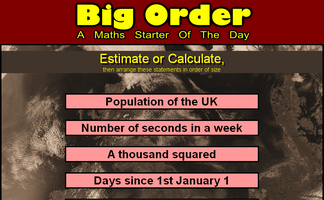Estimate or calculate then put the large numbers in order of size.How many breaths have you taken in your lifetime?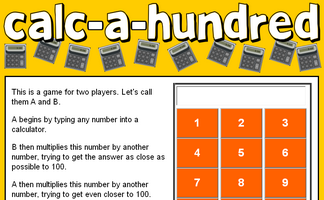A game for two players requiring a calculator and thinking skills.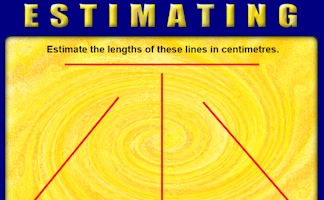Estimate the lengths of four lines then add your answers together.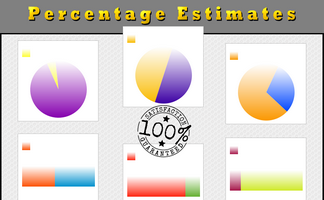Estimate the percentages of full circles and rectangles the sectors represent.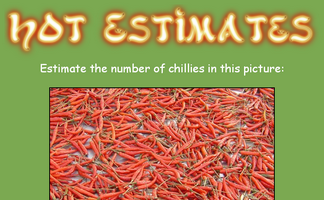Estimate the number of chillies in the photograph.Estimate the distances shown on this photograph of the moon's surface.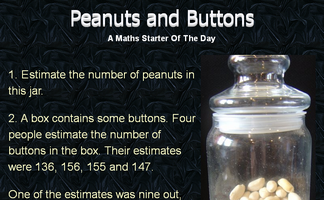Two questions involving estimating a quantity.An exercise in estimating what the sectors of a pie chart represent.Stand at the point between the classroom walls to represent a given number.Either estimate the lengths of the red lines or, if you know how, calculate how long they are.Estimate then work out the period of time equal to the given number of seconds.

### Search

The activity you are looking for may have been classified in a different way from the way you were expecting. You can search the whole of Transum Maths by using the box below.

Have today's Starter of the Day as your default homepage. Copy the URL below then select
Tools > Internet Options (Internet Explorer) then paste the URL into the homepage field.

Set as your homepage (if you are using Internet Explorer)

Do you have any comments? It is always useful to receive feedback and helps make this free resource even more useful for those learning Mathematics anywhere in the world. Click here to enter your comments.For All: